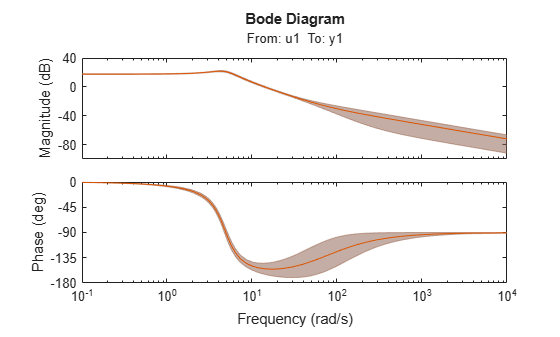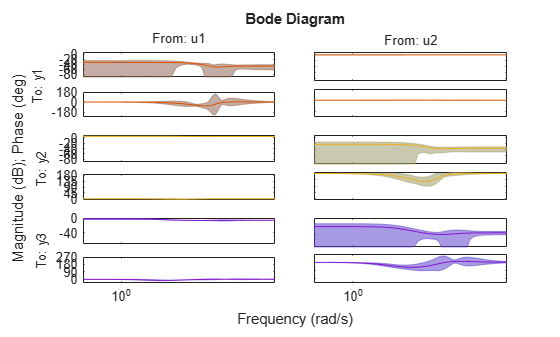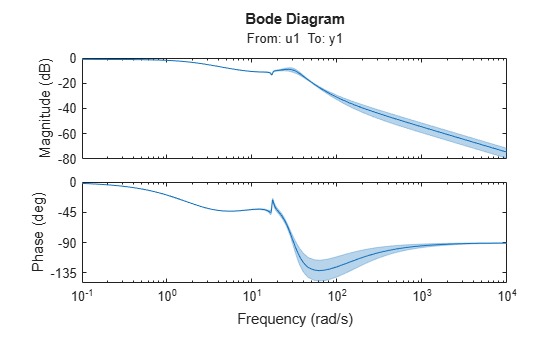# translatecov

Translate parameter covariance across model transformation operations

## Syntax

``newSys = translatecov(fcn,sys)``
``newSys = translatecov(fcn,Input1,...,InputN)``

## Description

example

````newSys = translatecov(fcn,sys)` transforms `sys` into `sysNew = fcn(sys)` and translates the parameter covariance of `sys` to the parameter covariance of the transformed model. `fcn` is a transformation function that you specify. The command computes the parameter covariance of `sys` using the Gauss Approximation formula. For more information, see Algorithms.Applying model transformations directly does not always translate the parameter covariance of the original model to that of the transformed model. For example, `d2c(sys)` does not translate the parameter covariance of `sys`. In contrast, `translatecov(@(x)d2c(x),sys)` produces a transformed model that has the same coefficients as `d2c(sys)` and has the translated parameter covariance of `sys`.```

example

````newSys = translatecov(fcn,Input1,...,InputN)` returns the model `sys_new = fcn(Input1,...,InputN)` and its parameter covariance. At least one of the `N` inputs must be a linear model with parameter covariance information.```

## Examples

collapse all

Convert an estimated transfer function model into state-space model while also translating the estimated parameter covariance.

Estimate a transfer function model.

```load iddata1 sys1 = tfest(z1,2);```

Convert the estimated model to state-space form while also translating the estimated parameter covariance.

`sys2 = translatecov(@(x)idss(x),sys1);`

If you convert the transfer function model to state-space form directly, the estimated parameter covariance is lost (the output of `getcov` is empty).

```sys3 = idss(sys1); getcov(sys3)```
```ans = [] ```

View the parameter covariance in the estimated and converted models.

```covsys1 = getcov(sys1); covsys2 = getcov(sys2);```

Compare the confidence regions.

```h = bodeplot(sys1,sys2); showConfidence(h,2);```The confidence bounds for `sys1` overlaps with `sys2`.

Concatenate 3 single-output models such that the covariance data from the 3 models combine to produce the covariance data for the resulting model.

Construct a state-space model.

```a = [-1.1008 0.3733;0.3733 -0.9561]; b = [0.7254 0.7147;-0.0631 -0.2050]; c = [-0.1241 0; 1.4897 0.6715; 1.4090 -1.2075]; d = [0 1.0347; 1.6302 0; 0.4889 0]; sys = idss(a,b,c,d,'Ts',0);```

Generate multi-output estimation data.

```t = (0:0.01:0.99)'; u = randn(100,2); y = lsim(sys,u,t,'zoh'); y = y + rand(size(y))/10; data = iddata(y,u,0.01); ```

Estimate a separate model for each output signal.

```m1 = ssest(data(:,1,:),2,'feedthrough',true(1,2), 'DisturbanceModel', 'none'); m2 = ssest(data(:,2,:),2,'feedthrough',true(1,2), 'DisturbanceModel', 'none'); m3 = ssest(data(:,3,:),2,'feedthrough',true(1,2), 'DisturbanceModel', 'none');```

Combine the estimated models while also translating the covariance information.

```f = @(x,y,z)[x;y;z]; M2 = translatecov(f, m1, m2, m3);```

The parameter covariance is not empty.

`getcov(M2, 'factors')`
```ans = struct with fields: R: [36x36 double] T: [24x36 double] Free: [90x1 logical] ```

If you combine the estimated models into one 3-output model directly, the covariance information is lost (the output of `getcov` is empty).

```M1 = [m1;m2;m3]; getcov(M1)```
```ans = [] ```

Compare the confidence bounds.

```h = bodeplot(M2, m1, m2, m3); showConfidence(h);```The confidence bounds for `M2` overlap with those of `m1`, `m2` and `m3` models on their respective plot axes.

Consider a closed-loop feedback model consisting of a plant and controller. Translate the parameter covariance of the plant to the closed-loop feedback model.

Estimate a plant as a fourth-order state-space model using estimation data `z1`.

```load iddata1 z1 Plant = ssest(z1,4);```

`Plant` contains parameter covariance information.

Create a controller as a continuous-time zero-pole-gain model with zeros, poles, and gain equal to -2, -10, 5, respectively.

`Controller = zpk(-2,-10,5);`

Define a transformation function to generate the closed-loop feedback state-space model.

`fcn = @(x,y)idss(feedback(x,y));`

Translate the parameter covariance of the plant to the closed-loop feedback model.

`sys_new = translatecov(fcn,Plant,Controller);`

`sys_new` contains the translated parameter covariance information.

Plot the frequency-response of the transformed model `sys_new`, and view the confidence region of the response.

```h = bodeplot(sys_new); showConfidence(h);```The plot shows the effect of the uncertainty in `Plant` on the closed-loop response.

## Input Arguments

collapse all

Model transformation function, specified as a function handle with one of the following syntaxes.

• Single input — `sysNew = fcn(sys)`

• Multiple inputs — `sysNew = fcn(Input1,..InputN)`

If `fcn` has one input argument, the input must be a linear identified model with parameter covariance information. Typical single-input transformation operations include:

• Convert to different model type, such as `idpoly` and `idss`. For example, ```fcn = @(x)idpoly(x)``` converts the model `x` to a polynomial model.

• Change model sample time using, for example, `c2d` or `d2c`. For example, ```fcn = @(x)c2d(x,Ts)``` converts the continuous-time model `x` to a discrete-time model with sample time `Ts`.

If `fcn` has multiple input arguments, at least one input argument must be an identified model with parameter covariance information (see `sys`). Typical multi-input operations include merging and concatenating of multiple models. For example, `fcn = @(x,y)[x,y]` performs horizontal concatenation of the models `x` and `y`.

Linear identified model to use as input for transformation function `fcn`, returned as one of the following identified model objects.

Multiple input arguments to the translation function `fcn`. At least one of the inputs must be a linear identified model with parameter covariance information. The other inputs can be any MATLAB data type.

## Output Arguments

collapse all

Model resulting from transformation, which includes parameter covariance information, returned as an identified model object.

To view the translated parameter covariance, use `getcov`.

## Tips

• If you obtained `sys` through estimation and have access to the estimation data, you can use zero-iteration update to recompute the parameter covariance. For example:

```load iddata1 m = ssest(z1,4); opt = ssestOptions opt.SearchOptions.MaxIterations = 0; m_new = ssest(z1,m2,opt) ```

You cannot run a zero-iteration update for some model and data type combinations, such as a continuous-time `idpoly` model using time-domain data.

Also, you cannot run a zero-iteration update if the `MaxIterations` option, which depends on the `SearchMethod` option, is not available.

## Algorithms

`translatecov` uses numerical perturbations of individual parameters of `sys` to compute the Jacobian of `fcn(sys)` parameters with respect to parameters of `sys`. `translatecov` then applies Gauss Approximation formula to translate the covariance, where J is the Jacobian matrix. This operation can be slow for models containing a large number of free parameters.

## Version History

Introduced in R2012b